# SSC JE Electrical Important MCQ Part 21

SSC JE Electrical Important MCQ PDF Part 21

1.In Figure if i = cos 4t and v = sin 4t, the element isA. a resistor

B. a capacitor

C. an inductor

D. none of these

(Given i = cos 4t and v = sin 4t

We know cos x=sin (x+90⁰)

cos (4t)=sin (4t+90⁰)

So i = sin (4t+90⁰)—-(1)

v = sin 4t—-(2)

By equation (1) & (2) it clear voltage lags the current by 90o.

In purely capacitive circuit the alternating voltage lags the current by 90o.So element is a capacitor)

2.Voltage across 3μF capacitor is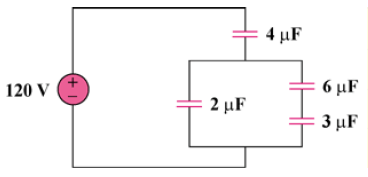A. 60V

B. 20V

C. 40V

D. 120V

(3μF is in series with 6μF=3×6/(9) =2μF

Now this 2μF is in parallel with 2μF=2+2=4 μF

v4μF = 1/2 x 120 = 60V

v2μF = 60V

v3μF =6*60/(6+3)= 40V)

3.Ceq for circuit in FigA. 1C

B. 2C

C. 4C

D. 12C

(Ceq of both parallel branches=C+C=2C,Now equivalent branches in series

So 1/Ceq =1/2C+1/2C=1/C or Ceq=1 C)

4.Leq in of the circuits in Fig.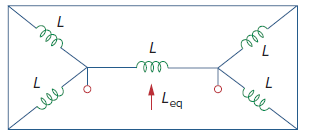A. 50 L

B. 500 L

C. 0.5 L

D. 5 L

(L//L = 0.5L

So L//L + L//L = L

Leq = L//L = 0.5L)

5.The charge entering a certain element is shown in Fig.The value of current at t = 1 ms is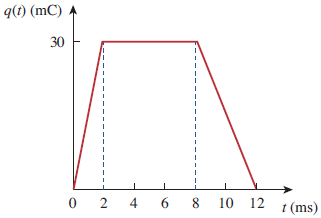A. 30 A

B. 0 A

C. -15 A

D. 15 A

(At t = 1ms, i=dq/dt=(30-0)/(2-0)=30/2=15 A

Note-dq,dt is the change in value in curve area for example At t = 10ms, i=dq/dt=(0-30)/(12-8)=-30/4=-7.5 A )

6.The V₀ in the circuit of Fig isA. 18 V

B. 6 V

C. 20 V

D. 10 V

(Since Σ p = 0

-30×6 + 6×12 + 3V₀ + 28 + 28×2 – 3×10 = 0

72 + 84 + 3V₀ = 210 or 3V₀ = 54

V₀= 18 V)

7.Figure shows the power consumption of a certain household in 1 day. The total energy consumed in kWh, will be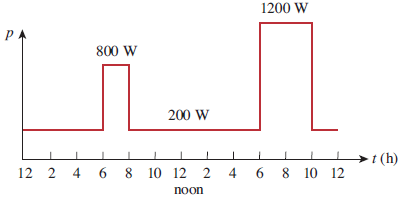A. 10 kWh

B. 100 kWh

C. 1.0 kWh

D. 20 kWh

Answer : A (Energy = Σpt = 200 x 6 + 800 x 2 + 200 x 10 + 1200 x 4 + 200 x 2= 10 kWh)

8.The number of branches and nodes in the circuit of Fig is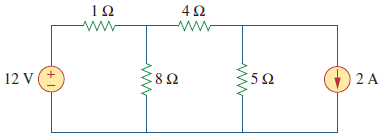A.6 branches and 4 nodes

B.4 branches and 2 nodes

C.5 branches and 4 nodes

D.2 branches and 2 nodes

9.Value of currents i₁,i₂, and i₃ in the circuit shown in FigA. i₁=4 A,i₂=1 A,i₃=3 A

B. i₁=-4 A,i₂=1 A,i₃=-3 A

C. i₁=4 A,i₂=-1 A,i₃=-3 A

D. i₁=4 A,i₂=-1 A,i₃=3 A

(At node A, 8 = 12 + i₁ or i₁ = – 4A

At node C, 9 = 8 + i₂ or  i₂ = 1A

At node D, 9 = 12 + i₂ or i₂ = -3A)

10.Consider the Wheatstone bridge shown in Fig.,What will be vab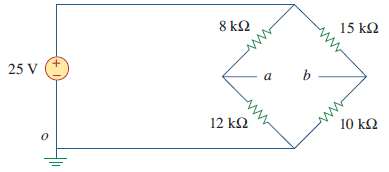A. -5 V

B. 10 V

C. 15 V

D. 5 V

(Using voltage division

Va =12*25/(12+8)=15 V

Vb =10*25/(10+15)=10 V

Vab = 15 − 10=5 V)

11.Standardization of potentiometers is done in order that, they become

A. Accurate

B. Precise

D. Accurate and precise

12. A potentiometer is basically a

A. Deflection type instrument

B. Null type instrument

C. Deflection as well as null type instrument

D. A digital instrument

13.Electronic null detectors are

A .Less sensitive

B. More sensitive.

C. More expensive.

D.Of excellent sensitivity, rugged construction and fairly expensive

14.The Recorder used for plotting B-H curves for magnetic materials

A. LVDT type recorder

B. Circular chart recorder

C. X-Y recorder

D. Potentiometer type recorder

15.Galvanometer type recorder use

A. Vibration galvanometer

B. Ballistic galvanometer

C. D’Arsonval galvanometer

D. Tangent galvanometer

16.The piezoelectric crystal sensitivity is defined as

A. Voltage developed per unit stress

B. Field developed per unit stress

C.Voltage developed per unit force

D.Field developed per unit force

17.Which one of the following is a passive transducer

A. Piezoelectric

B. Thermocouple

C. Photovoltaic cell

D. LVDT

Answer : D (Passive transducer is a device which converts the given non-electrical energy into electrical energy by external force. Resistance strain gauge, Differential Transformer/LVDT are the examples for the Passive transducers.)

18.Which of the following errors can arise, as a result of mistake in reading, parallax improper instrument location and inadequate lighting

A. Construction errors

B. Transmission errors

C. Observation errors

D. Translation errors

19.Relation between standard deviation and variance is

A. V = √σ

B. V = σ²

C. V = 1 / σ

D. V = 1/ √σ

20.A 0-1 mA meter has a sensitivity of

A.1 kΩ/V

B. 1 mA

C. 1 k Ω

D. 1000 A

Answer : A (sensitivity S=1/Ifsd=1/1 mA=1 kΩ/V)